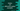# Python Set difference_update explanation with an example## Python Set difference_update :

In this tutorial, we will learn about the differenceupdate_ method of python set.

### Definition of difference_update :

The difference between two set SetA and SetB is a new set that holds only the elements of SetA and not of SetB. SetA - SetB is used to indicate the difference.

For two set SetA and SetB, the differenceupdate_ method will convert SetA to SetA - SetB. In other words, this method is an alternative way to find out the difference between the two sets.

### Syntax of difference_update :

The syntax is as below :

``SetA.difference_update(SetB)``

SetA and SetB are two different sets. This method call will update the SetA values with SetA - SetB. It will return None. Only the value of SetA will change.

SetB will remain unchanged.

### Example Program :

``````#1
setA = set()
setB = set()

#2
setA_length = int(input(“Enter the size of the first set : “))
setB_length = int(input(“Enter the size of the second set : “))

#3
print(“\n”)
print(“Enter values for the first set one by one : \n”)
for i in range(setA_length):
e = int(input(“Enter value {} : “.format(i+1)))

#4
print(“\n”)
print(“Enter values for the second set one by one : \n”)
for i in range(setB_length):
e = int(input(“Enter value {} : “.format(i+1)))

#5
print(“\nBefore : “)
print(“SetA : {} “.format(setA))
print(“SetB : {} “.format(setB))

#6
setA.difference_update(setB)

#7
print(“\nAfter : “)
print(“SetA : {} “.format(setA))
print(“SetB : {} “.format(setB))``````

### Explanation :

The commented numbers in the above program denote the step numbers below :

1. Create two empty set setA and setB.
2. Ask the user to enter the size of the first and the second set. Read the values and store them in setAlength_ and setBlength_ variables.
3. Read the inputs for the first set one by one using one for loop.
4. Similarly, read the inputs for the second set one by one using one for loop.
5. Print values of both set to the user.
6. Call differenceupdate_ method on setA and setB.
7. Print values of both set to the user again after the differenceupdate_ method call.

### Sample Output :

``````Enter the size of the first set : 3
Enter the size of the second set : 2

Enter values for the first set one by one :

Enter value 1 : 1
Enter value 2 : 2
Enter value 3 : 3

Enter values for the second set one by one :

Enter value 1 : 1
Enter value 2 : 2

Before :
SetA : {1, 2, 3}
\$ python3 example.py
Enter the size of the first set : 3
Enter the size of the second set : 2

Enter values for the first set one by one :

Enter value 1 : 1
Enter value 2 : 2
Enter value 3 : 3

Enter values for the second set one by one :

Enter value 1 : 1
Enter value 2 : 2

Before :
SetA : {1, 2, 3}
SetB : {1, 2}

After :
SetA : {3}
SetB : {1, 2}``````

### Conclusion :

differenceupdate_ method comes in handy if you want to find out the difference between two sets and the original sets are not required later. Instead of creating a new variable and place the new values in it, we can use differenceupdate_ and put the difference elements in the first set. Try to run the example shown above and drop one comment below if you have any queries.